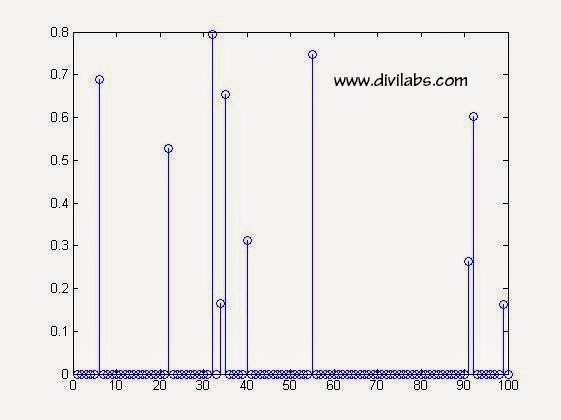# Generate Row & column vector from Any Matrix in Matlab

Converting from a matrix to its equivalent row or column vector is very easy in MATLAB. This is particularly useful in communication where the data or here matrix has to be send serially. The matrix will be converted to a n x 1 or 1 x n vector & sent element by element. This process is often called vectorization. It has many applications in Sparse Signal Recovery & image processing also.

Suppose you have a random 5 x 5 matrix generated by rand(5,5); function  stored in variable 'x'.
In our case it is,
>> x=rand(5,5)

>> x=
0.3197    0.1639    0.6798    0.0195    0.9360
0.6711    0.4944    0.3285    0.6075    0.4886
0.5240    0.7647    0.5383    0.5388    0.6976
0.6078    0.3008    0.8378    0.2263    0.5166
0.7603    0.8389    0.2430    0.9199    0.2852

Now to convert the matrix 'x' to a column vector we just need one command.
i.e.,
>> y=x(:) %suppose we store the resultant column vector in variable named 'y'
That will throw the result,
>> y =
0.3197
0.6711
0.5240
0.6078
0.7603
0.1639
0.4944
0.7647
0.3008
0.8389
0.6798
0.3285
0.5383
0.8378
0.2430
0.0195
0.6075
0.5388
0.2263
0.9199
0.9360
0.4886
0.6976
0.5166
0.2852

& >> size(y)=  [25   1] %column vector

Now to convert the matrix 'x' to a row vector we need only slight modification of previous command command.
i.e.,
>> z=x(:)' %suppose we store the resultant column vector in variable named 'z'

That will throw the result,
>>  z =
Columns 1 through 9
0.3197    0.6711    0.5240    0.6078    0.7603    0.1639    0.4944    0.7647    0.3008

Columns 10 through 18
0.8389    0.6798    0.3285    0.5383    0.8378    0.2430    0.0195    0.6075    0.5388

Columns 19 through 25
0.2263    0.9199    0.9360    0.4886    0.6976    0.5166    0.2852

& >> size(z)=  [1    25] %row vector

NOTE: It might be an important point to note that MATLAB while vectorization (or while converting matrix to a row or column vector) reads the elements column wise not row wise, which we usually learn in our schools. For doing that in row wise ways you must take first the transpose of the original matrix & then perform above operations.

i.e.,
x=rand(5,5)
b=x'; %taking transpose to convert columns into rows & storing in a new variable 'b'

y=b(:); %Column vector when read column wise of original 'x' & stored in 'y'

z=b(:)'; %Column vector when read column wise of original 'x' & stored in 'z'

# Generate a Random Sparse Signal Vector Using Randperm Function in MATLAB

Yes Matlab does have an inbuilt sparse data type & related function, which can be used very easily to generate a random sparse Vector. ( See :Creating & Using Sparse Matrix & Vectors in MATLAB - Concepts & Examples )
But there are other ways also in which you can generate it. One of them is through randperm() function of matlab.
To generate in this way it involves a little trick & some understanding upon how the Matlab's variable/vector index works & how randperm function works.

MATLAB Code for Random Sparse Signal Vector Using Randperm Function Will look Like this:

clc
clear all
close all
n=100;  %generation 100x1 vector sparse
k=10;
%Out of 'n' elements 'k' are non zero
xorg=zeros(n,1);
%initialization of the sparse vector as a zero vector 'xorg'
idx=randperm(n);  %we are calculating the index of first 'k' non zero elements through this step
xorg(idx(1:k))=rand(k,1); %take first 'k' elements of 'idx' & fill those index with a random value
stem(xorg) %plot the sparse vector thus generated

NOTE: # initializing by xorg=zeros(n,1); is necessary since if you are not doing this, you will generate x(org) in each run, with a different dimension.
# instead of rand(k,1) one can use randn(k,1) also.

Sample Run Of the Above MATLAB's Code:Random Sparse Signal Vector Using Randperm Function in MATLAB
How the Code Works?

** xorg=zeros(n,1); -> This command generate a 'n x 1' column vector of all elements being zero.

** idx=randperm(n); -> This command will generate a '1 x n' vector in which the elements are distributed randomly between 1 to n  (including this also). There is no repetition of any number between 1 to n, i.e., every element will be unique in this vector.

e.g., in the above it is,
idx = 99    32    40    22    34    92    91    35     6    55     3    96    68    16    69 & so on

** xorg(idx(1:k))=rand(k,1); -> idx(1:k) will select first 'k' elements from 'idx' here in this example it will be  99    32    40    22    34    92    91    35     6    55 & take them to select those index of 'xorg'. Then set those index value of 'xorg' to some random generated value ranging from 0 to 1 (since we are using rand( ) function & using  stem(xorg); -> plot the generated sparse signal

You can verify that index number 99    32    40    22    34    92    91    35     6    55 are non zero & others are all zero.
In our execution, the result was.
xorg=

0
0
0
0
0
0.689214503140008
% 6th index
0
0
0
0
0
0
0
0
0
0
0
0
0
0
0
0.528533135506213
% 22nd index
0
0
0
0
0
0
0
0
0
0.794284540683907
%32nd index
0
0.165648729499781
%34th index
0.654079098476782 %35th index
0
0
0
0
0.311215042044805
%40th index
0
0
0
0
0
0
0
0
0
0
0
0
0
0
0.748151592823710
%55th index
0
0
0
0
0
0
0
0
0
0
0
0
0
0
0
0
0
0
0
0
0
0
0
0
0
0
0
0
0
0
0
0
0
0
0
0.262971284540144
%91th index
0.601981941401637
%92nd index
0
0
0
0
0
0
0.162182308193243
%99th index
0